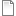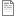# Paired t-test example

ModelId:
paired_ttest
SimileVersion:
5.x

This is an example of a paired t-test which is supposed to show the role of a null model in making statistical inference.

In this example there are two samples, each with 20 observations. We want to know if the sample means are statistically different.

The statistical question is:

What is the chance that the two samples are measurements of the same process, and the observed difference happened purely by chance?

To answer this question we could use a paired t-test. At its core the paired t-test has a null model.

The null model is that distribution of differences between the two samples is normal with a mean of zero and a variance equal to the observed variance. This model has assumptions. The two most obvious assumptions are 1/ The differences can be described with a normal distribution with the stated variance, 2/ The diferences are independent from one another.

Null models are normal described as a null hypothesis, with the assumptions tagged on the end. So the null hypothesis is that the mean difference between the samples is zero.

We want to know the probability of our observed difference if the null model had generated these data.

Since the normal distribution is relatively simple a paired t-test can calculate this probability exactly. But we could create the null model in simile, run the model many times, and then calculate the probability of generating a difference with the null model that is more extreme than our observations. With many runs of the null model (typically more than 1000), this probability is the p-value calculated by the paired t-test.

Any other statistical test can be performed like this. For example, an ANOVA is very similar, but rather than comparing means it compares variances. An ANOVA has an underlying null model, with assumptions. As the null models become more complicated the mathematics becomes harder. For complicated systems simulation is the only possibility. Methods that rely on computer simulation to some extent are Approximate Bayesian Computing (ABC), and Monte-Carlo methods.

Attached ar 3 files:

The model file is paired_ttest.sml

The interface for viewing the results is paired_ttest.shf

And the data used in this example are in the file t_test_data.txt

The model paired_ttest.sml calculates the two-sided and one-sided p-values from 1000 simulations of the null model. It also calculates the t-statistic and the dgrees of freedom which you can use to calculate the exact p-value with Students t-distribution.

AttachmentSizepaired_ttest.sml121.01 KBpaired_ttest.shf1.32 KBt_test_data.txt524 bytes
Model tags: